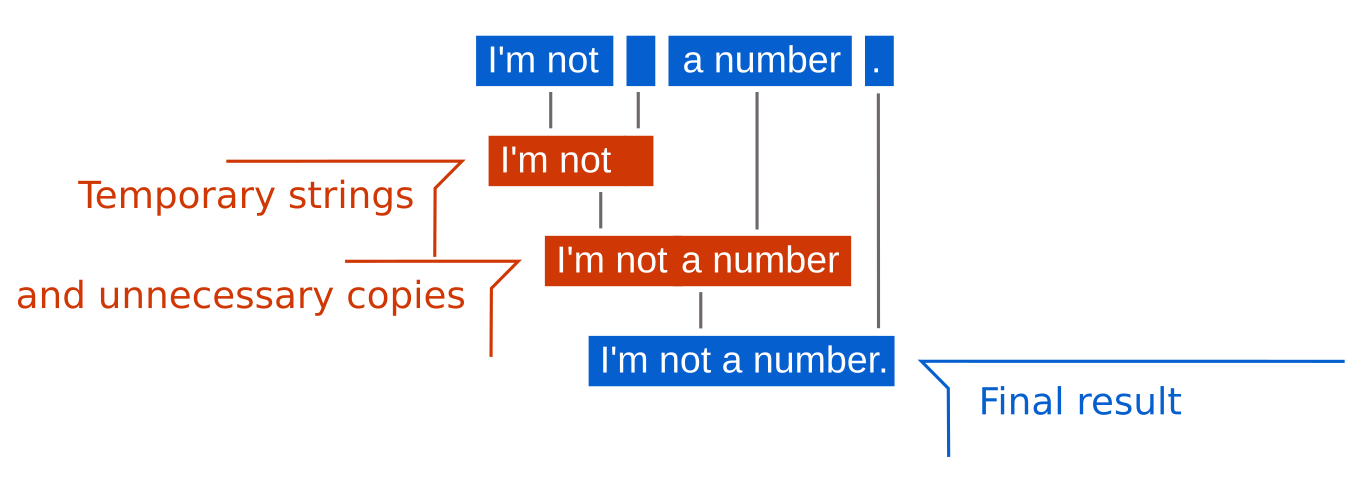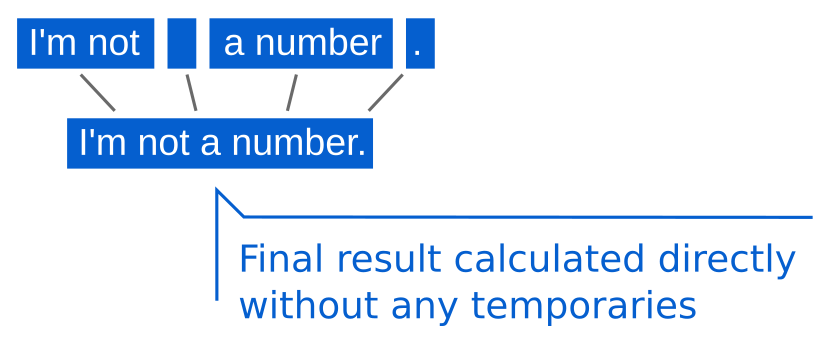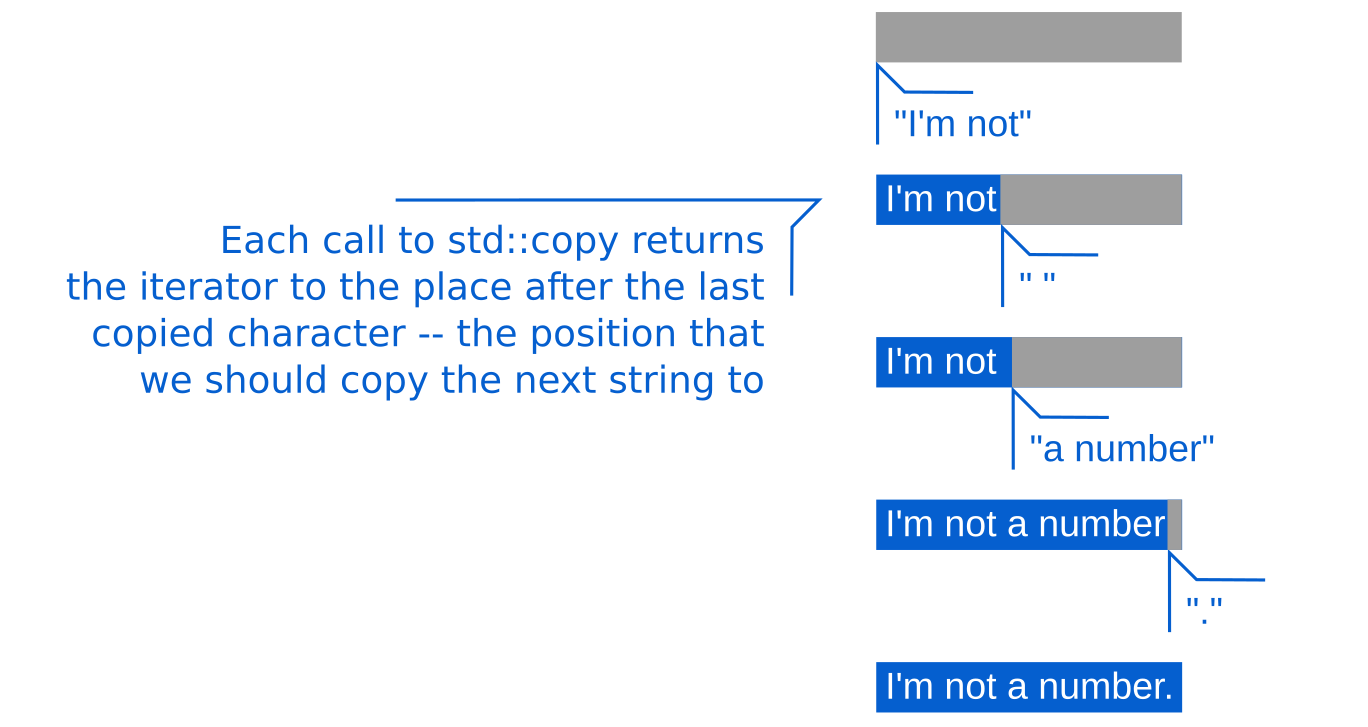# Efficient QString concatenation with C++17 fold expressions

In C++, we are accustomed to have the operator+to perform string concatenation, regardless of whether we are using the standard library (aka STL) or Qt. This allows us to write things like the following snippet:

``````QString statement{"I'm not"};
QString number{"a number"};
QString space{" "};
QString period{". "};
QString result = statement + space + number + period;``````

But this comes with a big drawback – unnecessary creation of temporary intermediate results. Namely, in the previous example, we have one temporary string to hold the result of the statement + spacepart of the expression, then that string gets concatenated with number which yields another temporary string. The second temporary string then gets concatenated with period which gives us the final result after which temporaries are then destroyed.

This means that we have almost as many unnecessary allocations and deallocations as we have calls to the operator+. Furthermore, we are copying the same data several times. For example, the contents of the statement string first get copied into the first temporary, then copied from the first temporary into the second, and then from the second temporary into the final result.Temporaries

This can be implemented in a much more efficient way by creating a string instance that preallocates the memory needed for storing the final result, and then consecutively calling the QString::appendmember function to append each of the strings we want to concatenate one by one:

``````QString result;
result.reserve(statement.length() + number.length() + space.length() + period.length();
result.append(statement);
result.append(number);
result.append(space);
result.append(period);``````Temporaries

Alternatively, we could use QString::resizeinstead of QString::reserve and then use std::copy(or std::memcpy) to copy the data into it (we will see how to use std::copy for string concatenation later). This would likely improve the performance a bit (depends on the compiler optimizations) because QString::append needs to check whether the string’s capacity is large enough to contain the resulting string. The std::copyalgorithm does not have this unnecessary extra check which can give it a slight edge.

Both of these approaches are significantly more efficient than using the operator+, but it would be annoying to have to write code like this every time we want to concatenate a few strings.

## The std::accumulate algorithm

Now, before we continue to how Qt solves this problem now, and a possible way to improve this in Qt 6 with the nice features we got in C++17, we need to cover one of the most important and most powerful algorithms from the standard library – the std::accumulate algorithm.

Imagine we gave a sequence (for example aQVector) of strings that we want to concatenate instead of having them in separate variables.

With std::accumulate, string concatenation would look like this:

``````QVector<QString> strings{ . . . };
std::accumulate(strings.cbegin(), strings.cend(), QString{});``````

The algorithm does what you’d expect in this example – it starts with an empty QString and adds each of the strings from the vector to it, thus creating a concatenated string.

This would be as inefficient as our original example of using the operator+ for concatenation since std::accumulate uses the operator+ internally by default.

In order to optimize this implementation like in the previous section, we can just use std::accumulate just to calculate the size of the resulting string instead of doing the whole concatenation with it:

``````QVector<QString> strings{ . . . };
QString result;
result.resize(
std::accumulate(strings.cbegin(), strings.cend(),
0, [] (int acc, const QString& s) {
return s.length();
}));``````

This time, the std::accumulate starts with the initial value 0and for each string sin the vector of strings, it adds the length of sto that initial value, finally returning the sum of lengths of all strings in the vector.

This is what std::accumulate means to most people – summation of some kind. But this is a rather simplistic way of looking at it.

In the first example, we did sumall the strings in the vector. But the second example is a bit different. We are not actually summing the elements of the vector. The vector contains QStrings and we are adding ints.

This is where the power of std::accumulate comes from – in the fact that we can pass a custom operation to it. The operation takes the previously accumulated value and one element of the source collection, and generates a new accumulated value. The first time std::accumulate calls this operation, it will pass it the initial value as the accumulator, and the first element of the source collection. It will take the result and pass it to the next call of the operation along with the second element of the source collection. This will be repeated until the whole source collection is processed, and the algorithm will return the result of the final operation call.

As seen in the previous code snippet, the accumulator does not even need to be of the same type as the values in the vector. We had a vector of strings while the accumulator was an integer.

We can leverage this to do something more fun.

The aforementioned std::copy algorithm takes a sequence of items that need to be copied (as a pair of input iterators) and the destination (as an output iterator) where the items should be copied to. It returns the iterator pointing to the element after the last copied item in the destination collection.

This means that if we copy the data of one source string into the destination string using std::copy, we’re going to get the iterator pointing to the exact location where we should copy the second string’s data to.

So, we have a function that takes a string (as a pair of iterators) and one output iterator, and gives us a new output iterator. This kinda looks like something we can use as the operation for std::accumulate to implement efficient string concatenation with:

``````QVector<QString> strings{ . . . };
QString result;
result.resize( . . . );

std::accumulate(strings.cbegin(), strings.cend(), result.begin(),
[] (const auto& dest, const QString& s) {
return std::copy(s.cbegin(), s.cend(), dest);
});``````

The first invocation of std::copy will copy the first string to the destination defined by result.begin(). It will return the iterator in the resultstring just after the last copied character, and that is where the second string from the vector will be copied to. The third string will be copied after that, and so on.Temporaries

In the end, we get a concatenated string.

## Recursive expression templates

We can now get back to the efficient string concatenation using operator+ in Qt.

``QString result = statement + space + number + period;``

We’ve seen that the problem with string concatenation stems from the fact that C++ evaluates the previous expression in steps – by calling the operator+several times where each call returns a new instance of QString.

While we can not change the way C++ evaluates this, we can use a technique called expression templatesto delay the actual resulting string calculation until the whole expression is defined. This can be done by changing the return type of operator+ not to be a QString, but some custom type that just stores the strings that should be concatenated without actually performing the concatenation.

In fact, this is exactly what Qt does since 4.6 if you activate the fast string concatenation. Instead of returning a QString the operator+ will return an instance of a hidden class template called QStringBuilder. The QStringBuilderclass template is just a dummy type that holds references to the arguments passed to the operator+.

Basically, a more complex version of the following:

``````template <typename Left, typename Right>
class QStringBuilder {
const Left& _left;
const Right& _right;
};``````

When you concatenate several strings, you will get a more complex type where several QStringBuilders are nested into each other. Something like this:

``QStringBuilder<QString, QStringBuilder<QString, QStringBuilder<QString, QString>>>``

This type is just a complex way to say “I hold four strings that should be concatenated”.

When we request the QStringBuilderto be converted to a QString (for example, by assigning it to the QString result), it will first calculate the total size of all the contained strings, then it will allocate a QStringinstance of that size and, finally, it will copy the strings one by one into the resulting string.

Essentially, it will do exactly the same thing we did earlier, but it will be done automagically, without us having to lift a finger.

The problem with the current implementation of QStringBuilder is that it implements a container of an arbitrary number of strings through nesting. Each QStringBuilder instance can contain exactly two items – be it strings or other QStringBuilder instances.

This means that all instances of QStringBuilderare a kind of a binary tree in which QStrings are leaf nodes. Whenever something needs to be done on the contained strings, the QStringBuilder needs to process its left sub-tree, then the right sub-tree recursively.

Instead of creating binary trees, we can use variadic templates (available since C++11, which was not available when QStringBuilder was invented). Variadic templates allow us to create classes and functions that have an arbitrary number of template arguments.

This means that, with the help of std::tuplewe can create a QStringBuilder class template that holds as many strings as we want:

``````template <typename... Strings>
class QStringBuilder {
std::tuple<Strings...> _strings;
};``````

When we get a new string to add to theQStringBuilder, we can just addit to the tuple using std::tuple_cat which concatenates two tuples (we’ll be using operator% instead of operator+ as this operator is also supported by QStringand QStringBuilder):

``````template <typename... Strings>
class QStringBuilder {
std::tuple<Strings...> _strings;

template <typename String>
auto operator%(String&& newString) &&
{
return QStringBuilder<Strings..., String>(
std::tuple_cat(_strings, std::make_tuple(newString)));
}
};``````

## Fold expressions

This is all and well, but the question is how do we process the variadic template parameter packs (the ... part of Strings).

With C++17, we got a new construct for processing parameter packs called fold expressions.

The general form of fold expressions look like this (the operator+ can be replaced with some other binary operator like *, %…):

``(init + ... + pack)``

or:

``(pack + ... + init)``

The first variant is called a left fold expression treats the operation as left-associative, and the second one is called a right fold expression because it treats the operation as right-associative.

If we wanted to concatenate strings in a template parameter pack using a fold expression, we could do it like so:

``````template <typename... Strings>
auto concatenate(Strings... strings)
{
return (QString{} + ... + strings);
}``````

This would first call the operator+ on the initial value QString{} and the first element of the parameter pack. Then, it would call the operator+ on the result of the previous call and the second element of the parameter pack. And so on until all the elements have been processed.

This sounds familiar, doesn’t it?

We saw the same behavior with std::accumulate. The only difference is that the std::accumulate algorithm operates on runtime sequences of data (vectors, arrays, lists, etc.) while the fold expressions operate on compile-time sequences, that is, variadic template parameter packs.

We can follow the same steps for optimizing the previous concatenation implementation that we had with std::accumulate. First, we need to calculate the sum of all string lengths. This is quite simple With fold expressions:

``````template <typename... Strings>
auto concatenate(Strings... strings)
{
const auto totalSize = (0 + ... + strings.length());
. . .
}``````

When the fold expression expands the parameter pack, it will get the following expression:

``0 + string1.length() + string2.length() + string3.length()``

So, we got the size of the resulting string. We can now proceed on to allocating a string sufficiently large for the result, and append the source strings one by one to it.

As previously mentioned, the fold expressions work with C++’s binary operators. If we want to execute a function for each element in the parameter pack, we can use one of the strangest operators in C and C++ – the comma operator.

``````template <typename... Strings>
auto concatenate(Strings... strings)
{
const auto totalSize = (0 + ... + strings.length());
QString result;
result.reserve(totalSize);

(result.append(strings), ...);

return result;
}``````

This will call append for each of the strings in the parameter pack giving us the concatenated string.

## Custom operators with fold expressions

The second approach we took with std::accumulate was a bit more complex – we had to provide a custom operation for accumulation. The accumulator was the iterator in the destination collection which denoted where we should copy the next string to.

If we want to have a custom operation with fold expressions, we need to create a binary operator. The operator, just like the lambda we passed to std::accumulate, needs to take one output iterator and one string, it needs to call std::copy to copy the string data to that iterator, and it needs to return a new iterator pointing to the element after the last copied character.

For this, we can redefine operator<<:

``````template <typename Dest, typename String>
auto operator<< (Dest dest, const String& string)
{
return std::copy(string.cbegin(), string.cend(), dest);
}``````

With this implemented, the fold expression that copies all strings into the destination buffer becomes quite simple. The initial value is the begin iterator of the destination buffer, and we <<each of the strings in the parameter pack to it:

``````template <typename... Strings>
auto concatenate(Strings... strings)
{
const auto totalSize = (0 + ... + strings.length());
QString result;
result.resize(totalSize);

(result.begin() << ... << strings);

return result;
}``````

## Fold expressions and tuples

We now know how to efficiently concatenate a collection of strings – be it a vector or a variadic template parameter pack.

The problem is that our QStringBuilder does not have either. It stores the strings inside of a std::tuple which is not an iterable collection, nor a parameter pack.

In order to work with fold expressions, we need parameter packs. Instead of the parameter pack containing strings, we can create a pack that contains a list of indices from 0 to n-1 which we can later use with std::getto access the values inside of the tuple.

This pack is easily created by using the std::index_sequence which represents a compile-time list of integers. We can create a helper function that accepts the std::index_sequence<Idx...> as its argument, and then use std::get<Idx>(_strings) to access strings from the tuple one by one from the fold expression.

``````template <typename... Strings>
class QStringBuilder {
using Tuple = std::tuple<Strings...>;
Tuple _strings;

template <std::size_t... Idx>
auto concatenateHelper(std::index_sequence<Idx...>) const
{
const auto totalSize = (std::get<Idx>(_strings).size() + ... + 0);

QString result;
result.resize(totalSize);

(result.begin() << ... << std::get<Idx>(_strings));

return result;
}
};``````

We just need to create a wrapper function that creates the index sequence for our tuple, and call the concatenateHelper function:

``````template <typename... Strings>
class QStringBuilder {
. . .

auto concatenate() const
{
return concatenateHelper(
std::index_sequence_for<Strings...>{});
}
};``````

## Wrap up

This post dealt only with the actual string concatenation. In order to apply this to the real QStringBuilder, we would need a few more things, and the implementation would become a bit overwhelming for reading as a blog post.

We would need to be careful with operator overloading – we would have to use std::enable_iflike the current QStringBuilder implementation to make it work all Qt’s concatenable types and not taint the global space with those operators.

It would also be beneficial to be able to handle temporaries passed to the string concatenation in a safer way as QStringBuilder stores only the references to the strings which, in the case of temporary strings, can easily become dangling references.